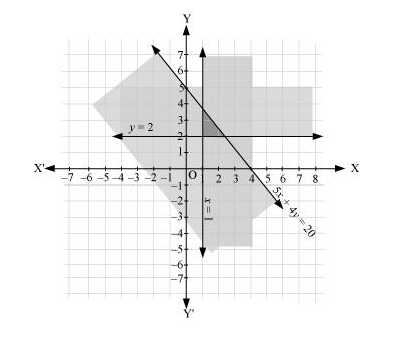# Solve the following system of inequalities graphically: 5x + 4y ≤ 20, x ≥ 1, y ≥ 2

Question:

Solve the following system of inequalities graphically: 5x + 4y  20, x  1, y  2

Solution:

$5 x+4 y \leq 20$  (1)

$x \geq 1 \ldots(2)$

$y \geq 2 \ldots(3)$

The graph of the lines, 5x + 4y = 20, x = 1, and y = 2, are drawn in the figure below.

Inequality (1) represents the region below the line, 5x + 4y = 20 (including the line 5x + 4y = 20). Inequality (2) represents the region on the right hand side of the line, x = 1 (including the line x = 1). Inequality (3) represents the region above the line, y = 2 (including the line y = 2).

Hence, the solution of the given system of linear inequalities is represented by the common shaded region including the points on the respective lines as follows.